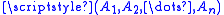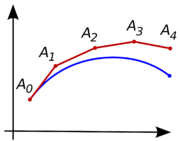xPolygonal chainEncyclopediaA polygonal chain, polygonal curve, polygonal path, or piecewise linear curve, is a connected series of line segment
Line segment
In geometry, a line segment is a part of a line that is bounded by two end points, and contains every point on the line between its end points. Examples of line segments include the sides of a triangle or square. More generally, when the end points are both vertices of a polygon, the line segment...

s. More formally, a polygonal chain P is a curve
Curve
In mathematics, a curve is, generally speaking, an object similar to a line but which is not required to be straight...

specified by a sequence
Sequence
In mathematics, a sequence is an ordered list of objects . Like a set, it contains members , and the number of terms is called the length of the sequence. Unlike a set, order matters, and exactly the same elements can appear multiple times at different positions in the sequence...

of pointscalled its vertices so that the curve consists of the line segments connecting the consecutive vertices.

In computer graphics
Computer graphics
Computer graphics are graphics created using computers and, more generally, the representation and manipulation of image data by a computer with help from specialized software and hardware....

a polygonal chain is called a polyline and is often used to approximate curved paths.

A simple polygonal chain is one in which only consecutive (or the first and the last) segments intersect and only at their endpoints.

A closed polygonal chain is one in which the first vertex coincides with the last one, or, alternatively, the first and the last vertices are also connected by a line segment. A simple closed polygonal chain in the plane is the boundary of a simple polygon
Simple polygon
In geometry, a simple polygon is a closed polygonal chain of line segments in the plane which do not have points in common other than the common vertices of pairs of consecutive segments....

. Often the term "polygon
Polygon
In geometry a polygon is a flat shape consisting of straight lines that are joined to form a closed chain orcircuit.A polygon is traditionally a plane figure that is bounded by a closed path, composed of a finite sequence of straight line segments...

" is used in the meaning of "closed polygonal chain".

In some cases it is important to draw a distinction between a polygonal area and a polygonal chain.

A polygonal chain is called monotone, if there is a straight line L such that every line perpendicular to L intersects the chain at most once. Every non trivial monotone polygonal chain is open. Compare with "Monotone polygon
Monotone polygon
In geometry, a polygon P in the plane is called monotone with respect to a straight line L, if every line orthogonal to L intersects P at most twice....

".

## Application and problemsPolygonal curves can be used to approximate other curves and boundaries of real-life objects.

• Polygon mesh
Polygon mesh
A polygon mesh or unstructured grid is a collection of vertices, edges and faces that defines the shape of a polyhedral object in 3D computer graphics and solid modeling...

- A 2-dimensional surface created by a connected network of polygons.
• Simplicial complex
Simplicial complex
In mathematics, a simplicial complex is a topological space of a certain kind, constructed by "gluing together" points, line segments, triangles, and their n-dimensional counterparts...

- a higher dimensional generalization limited to simplex
Simplex
In geometry, a simplex is a generalization of the notion of a triangle or tetrahedron to arbitrary dimension. Specifically, an n-simplex is an n-dimensional polytope which is the convex hull of its n + 1 vertices. For example, a 2-simplex is a triangle, a 3-simplex is a tetrahedron,...

facets
• Maurer rose
Maurer rose
In geometry, the concept of a Maurer rose was introduced by Peter M. Maurer in his article titled A Rose is a Rose.... A Maurer rose consists of some lines that connect some points on a rose curve.-Definition:...